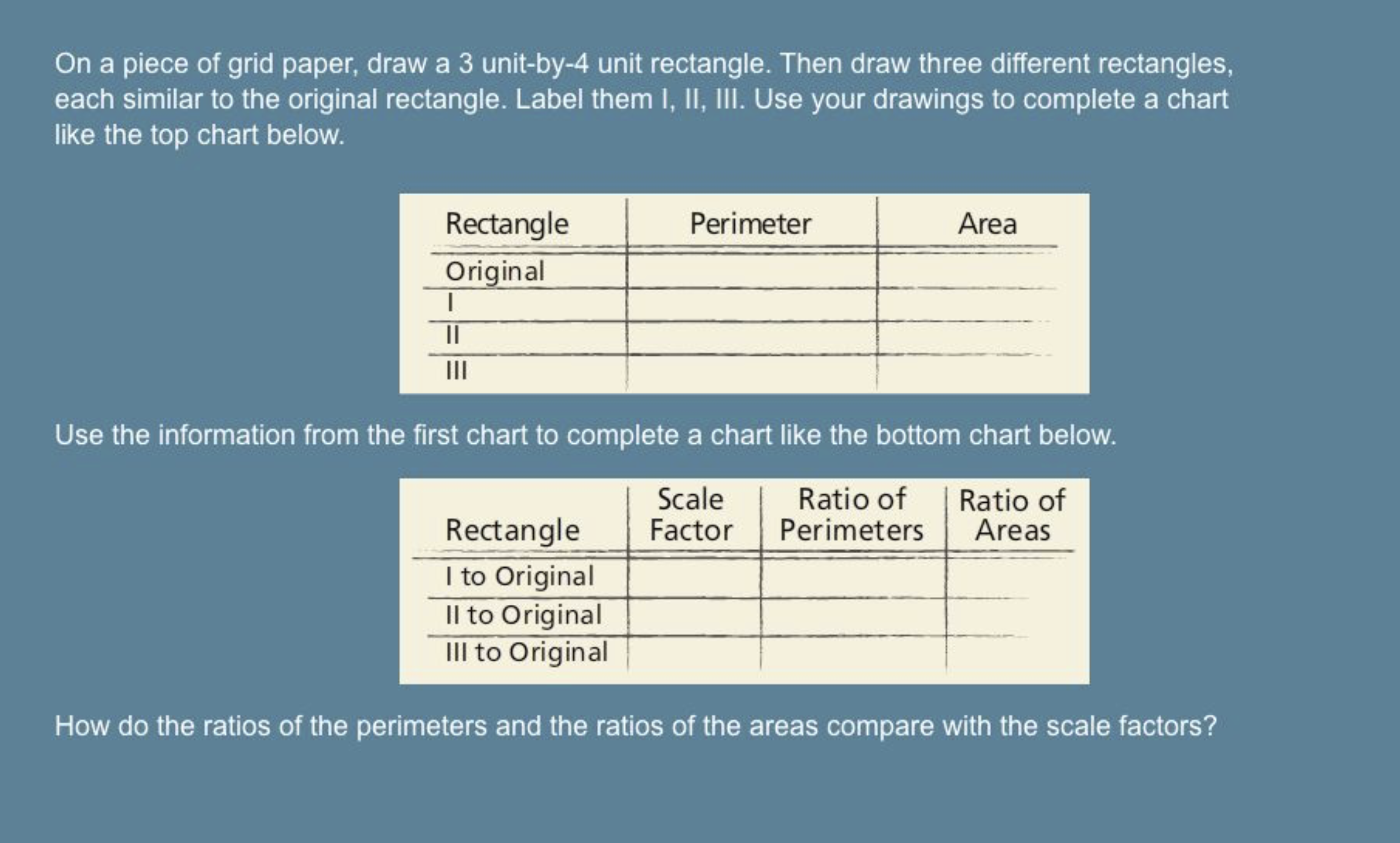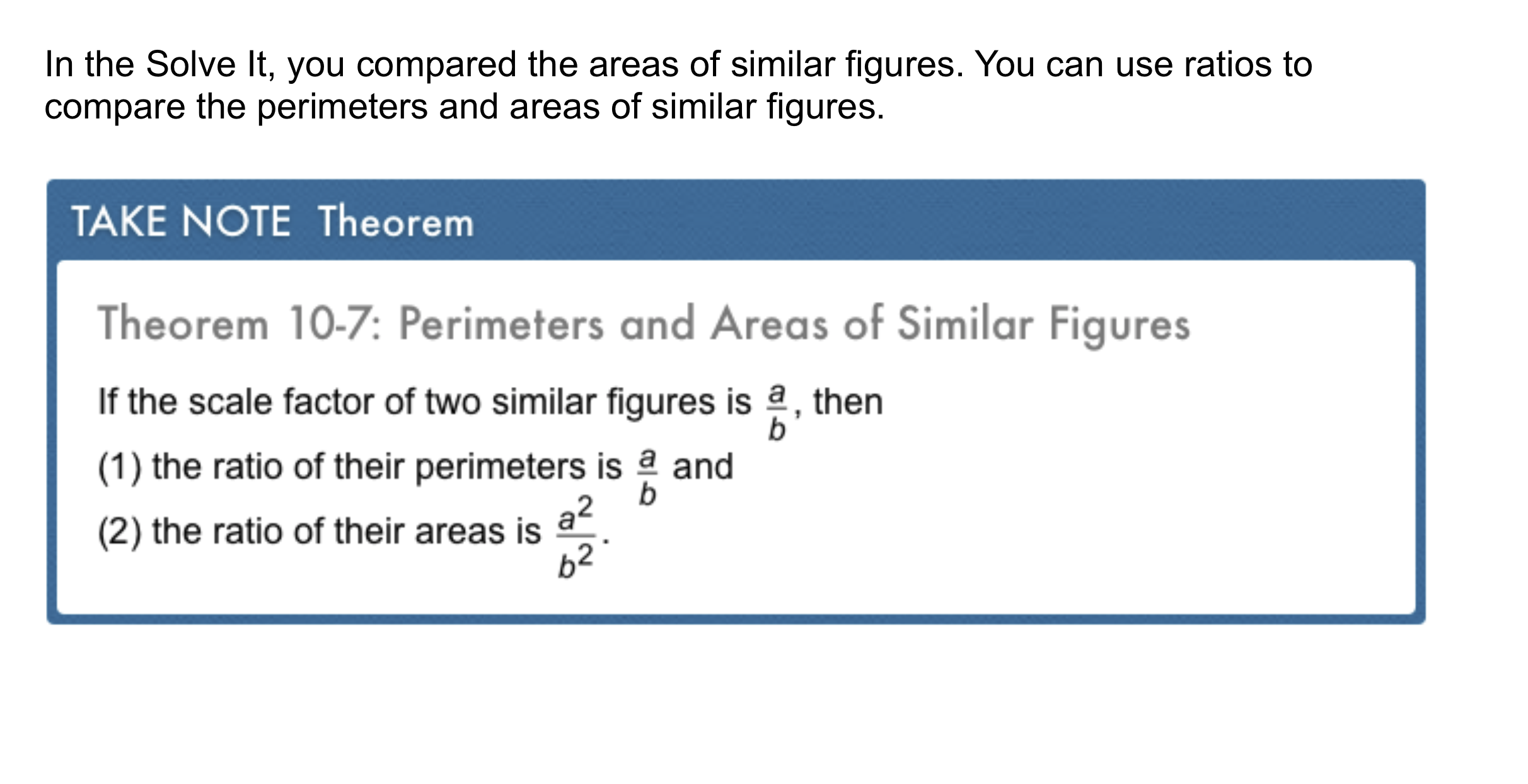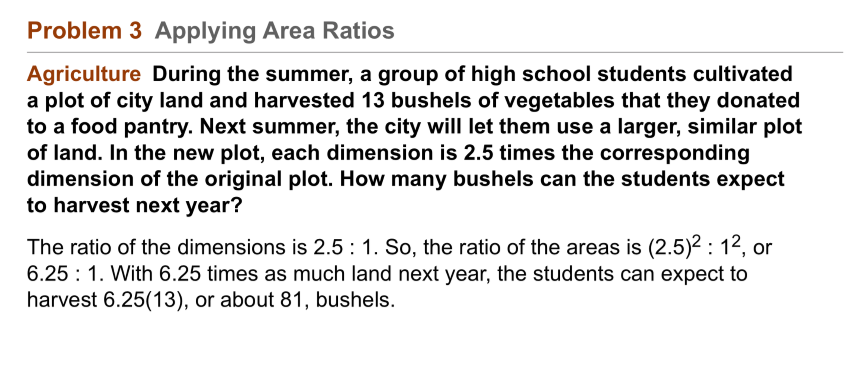Geometry 10-4 Perimeters of Areas of Similar Figures
starstarstarstarstarstarstarstarstarstar
by Matthew Richardson
| 24 Questions
Note from the author:
A complete formative lesson with embedded slideshow, mini lecture screencasts, checks for understanding, practice items, mixed review, and reflection. I create these assignments to supplement each lesson of Pearson's Common Core Edition Algebra 1, Algebra 2, and Geometry courses. See also mathquest.net and twitter.com/mathquestEDU.
The outlined content above was added from outside of Formative.
The outlined content above was added from outside of Formative.1
2
1
10 pts
Solve It! Complete the tables on the canvas using the text tool.
2
10 pts
Solve It! How do the ratios of the perimeters and the ratios of the areas compare with the scale factors?The outlined content above was added from outside of Formative.3
3
10 pts
Problem 1 Got It?
A
B
C
D4
4
10 pts
Problem 1 Got It?
A
B
C
D
The outlined content above was added from outside of Formative.5
5
10 pts
Problem 2 Got It?
A
B
C
D
The outlined content above was added from outside of Formative.
6
10 pts
Problem 3 Got It? The scale factor of the dimensions of two small pieces of window glass is 3 : 5. The smaller piece costs \$2.50. How much should the larger piece cost?

Enter your answer in this format: \$2.717
7
10 pts
Problem 3 Got It? Reasoning: In Problem 3, why is it important that each dimension is 2.5 times the corresponding dimension of the original plot? Explain.
The outlined content above was added from outside of Formative.8
8
10 pts
Problem 4 Got It?
A
B
C
D9
9
10 pts
A
B
C
D10
10
10 pts
A
B
C
D11
11
10 pts
A
B
C
D12
12
10 pts
A
B
C
D14
10 pts
Reasoning: The area of one rectangle is twice the area of a similar rectangle. What is the ratio of their perimeters? How do you know?
15
10 pts
Error Analysis: Your friend says that since the ratio of the perimeters of two polygons is ½, the area of the smaller polygon must be one half the area of the larger polygon. What is wrong with this statement? Explain.
16
10 pts
Compare and Contrast: How is the relationship between the areas of two congruent figures different from the relationship between the areas of two similar figures?17
10 pts
Review Lesson 10-3: Match each regular polygon with its area.
• 480 square units
• 690 square units
• 484 square units
• a square with apothem 11
• a regular pentagon with apothem 13.8 and side length 20
• an octagon with apothem 12 and side length 1018
18
10 pts
Review Lesson 10-3: Fill in the blank. Enter only a number. The area of the regular polygon is __?__ square inches.20
10 pts
Vocabulary Review: Categorize each statement based on whether it is always, sometimes, or never true.
• Corresponding angles of similar figures are congruent.
• Corresponding sides of similar figures are proportional.
• Always
• Sometimes
• Never
21
10 pts
Vocabulary Review: Identify the pairs of similar figures. Select all that apply.22
22
10 pts
Use Your Vocabulary: Which segment is NOT a radius of regular pentagon ABCDE?

23
10 pts
Use Your Vocabulary: Complete each sentence with are or are not.
• are
• are not
• The radii of a regular polygon __?__ congruent.
• The triangles formed by the radii and sides of regular pentagon ABCD __?__ congruent.
24
10 pts
Reflection: Math Success
Add to my formatives list

Formative uses cookies to allow us to better understand how the site is used. By continuing to use this site, you consent to the Terms of Service and Privacy Policy.